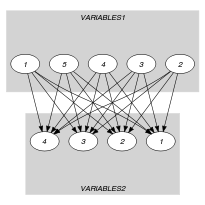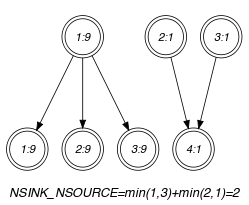## 5.368. soft_used_by_partition_var

Origin
Constraint

$\mathrm{𝚜𝚘𝚏𝚝}_\mathrm{𝚞𝚜𝚎𝚍}_\mathrm{𝚋𝚢}_\mathrm{𝚙𝚊𝚛𝚝𝚒𝚝𝚒𝚘𝚗}_\mathrm{𝚟𝚊𝚛}\left(𝙲,\mathrm{𝚅𝙰𝚁𝙸𝙰𝙱𝙻𝙴𝚂}\mathtt{1},\mathrm{𝚅𝙰𝚁𝙸𝙰𝙱𝙻𝙴𝚂}\mathtt{2},\mathrm{𝙿𝙰𝚁𝚃𝙸𝚃𝙸𝙾𝙽𝚂}\right)$

Synonym

$\mathrm{𝚜𝚘𝚏𝚝}_\mathrm{𝚞𝚜𝚎𝚍}_\mathrm{𝚋𝚢}_\mathrm{𝚙𝚊𝚛𝚝𝚒𝚝𝚒𝚘𝚗}$.

Type
 $\mathrm{𝚅𝙰𝙻𝚄𝙴𝚂}$ $\mathrm{𝚌𝚘𝚕𝚕𝚎𝚌𝚝𝚒𝚘𝚗}\left(\mathrm{𝚟𝚊𝚕}-\mathrm{𝚒𝚗𝚝}\right)$
Arguments
 $𝙲$ $\mathrm{𝚍𝚟𝚊𝚛}$ $\mathrm{𝚅𝙰𝚁𝙸𝙰𝙱𝙻𝙴𝚂}\mathtt{1}$ $\mathrm{𝚌𝚘𝚕𝚕𝚎𝚌𝚝𝚒𝚘𝚗}\left(\mathrm{𝚟𝚊𝚛}-\mathrm{𝚍𝚟𝚊𝚛}\right)$ $\mathrm{𝚅𝙰𝚁𝙸𝙰𝙱𝙻𝙴𝚂}\mathtt{2}$ $\mathrm{𝚌𝚘𝚕𝚕𝚎𝚌𝚝𝚒𝚘𝚗}\left(\mathrm{𝚟𝚊𝚛}-\mathrm{𝚍𝚟𝚊𝚛}\right)$ $\mathrm{𝙿𝙰𝚁𝚃𝙸𝚃𝙸𝙾𝙽𝚂}$ $\mathrm{𝚌𝚘𝚕𝚕𝚎𝚌𝚝𝚒𝚘𝚗}\left(𝚙-\mathrm{𝚅𝙰𝙻𝚄𝙴𝚂}\right)$
Restrictions
 $𝙲\ge 0$ $𝙲\le |\mathrm{𝚅𝙰𝚁𝙸𝙰𝙱𝙻𝙴𝚂}\mathtt{2}|$ $|\mathrm{𝚅𝙰𝚁𝙸𝙰𝙱𝙻𝙴𝚂}\mathtt{1}|\ge |\mathrm{𝚅𝙰𝚁𝙸𝙰𝙱𝙻𝙴𝚂}\mathtt{2}|$ $\mathrm{𝚛𝚎𝚚𝚞𝚒𝚛𝚎𝚍}$$\left(\mathrm{𝚅𝙰𝚁𝙸𝙰𝙱𝙻𝙴𝚂}\mathtt{1},\mathrm{𝚟𝚊𝚛}\right)$ $\mathrm{𝚛𝚎𝚚𝚞𝚒𝚛𝚎𝚍}$$\left(\mathrm{𝚅𝙰𝚁𝙸𝙰𝙱𝙻𝙴𝚂}\mathtt{2},\mathrm{𝚟𝚊𝚛}\right)$ $\mathrm{𝚛𝚎𝚚𝚞𝚒𝚛𝚎𝚍}$$\left(\mathrm{𝙿𝙰𝚁𝚃𝙸𝚃𝙸𝙾𝙽𝚂},𝚙\right)$ $|\mathrm{𝙿𝙰𝚁𝚃𝙸𝚃𝙸𝙾𝙽𝚂}|\ge 2$ $|\mathrm{𝚅𝙰𝙻𝚄𝙴𝚂}|\ge 1$ $\mathrm{𝚛𝚎𝚚𝚞𝚒𝚛𝚎𝚍}$$\left(\mathrm{𝚅𝙰𝙻𝚄𝙴𝚂},\mathrm{𝚟𝚊𝚕}\right)$ $\mathrm{𝚍𝚒𝚜𝚝𝚒𝚗𝚌𝚝}$$\left(\mathrm{𝚅𝙰𝙻𝚄𝙴𝚂},\mathrm{𝚟𝚊𝚕}\right)$
Purpose

For each integer $i$ in $\left[1,|\mathrm{𝙿𝙰𝚁𝚃𝙸𝚃𝙸𝙾𝙽𝚂}|\right]$, let $𝑁{\mathit{1}}_{i}$ (respectively $𝑁{\mathit{2}}_{i}$) denote the number of variables of $\mathrm{𝚅𝙰𝚁𝙸𝙰𝙱𝙻𝙴𝚂}\mathtt{1}$ (respectively $\mathrm{𝚅𝙰𝚁𝙸𝙰𝙱𝙻𝙴𝚂}\mathtt{2}$) that take their value in the ${i}^{th}$ partition of the collection $\mathrm{𝙿𝙰𝚁𝚃𝙸𝚃𝙸𝙾𝙽𝚂}$. $𝙲$ is the minimum number of values to change in the $\mathrm{𝚅𝙰𝚁𝙸𝙰𝙱𝙻𝙴𝚂}\mathtt{1}$ and $\mathrm{𝚅𝙰𝚁𝙸𝙰𝙱𝙻𝙴𝚂}\mathtt{2}$ collections so that for all $i$ in $\left[1,|\mathrm{𝙿𝙰𝚁𝚃𝙸𝚃𝙸𝙾𝙽𝚂}|\right]$ we have $𝑁{\mathit{2}}_{i}>0⇒𝑁{\mathit{1}}_{i}\ge 𝑁{\mathit{2}}_{i}$.

Example
$\left(\begin{array}{c}2,〈9,1,1,8,8〉,\hfill \\ 〈9,9,9,1〉,\hfill \\ 〈𝚙-〈1,2〉,𝚙-〈9〉,𝚙-〈7,8〉〉\hfill \end{array}\right)$

In the example, the values of the collections $〈9,1,1,8,8〉$ and $〈9,9,9,1〉$ are respectively associated with the partitions $𝚙-〈9〉$, $𝚙-〈1,2〉$, $𝚙-〈1,2〉$, $𝚙-〈7,8〉$, $𝚙-〈7,8〉$ and $𝚙-〈9〉$, $𝚙-〈9〉$, $𝚙-〈9〉$, $𝚙-〈1,2〉$. Since there is a correspondence between two pairs of partitions we must unset at least $4-2$ items (4 is the number of items of the $\mathrm{𝚅𝙰𝚁𝙸𝙰𝙱𝙻𝙴𝚂}\mathtt{2}$ collection). Consequently, the $\mathrm{𝚜𝚘𝚏𝚝}_\mathrm{𝚞𝚜𝚎𝚍}_\mathrm{𝚋𝚢}_\mathrm{𝚙𝚊𝚛𝚝𝚒𝚝𝚒𝚘𝚗}_\mathrm{𝚟𝚊𝚛}$ constraint holds since its first argument $𝙲$ is set to $4-2$.

Typical
 $𝙲>0$ $|\mathrm{𝚅𝙰𝚁𝙸𝙰𝙱𝙻𝙴𝚂}\mathtt{1}|>1$ $|\mathrm{𝚅𝙰𝚁𝙸𝙰𝙱𝙻𝙴𝚂}\mathtt{2}|>1$ $\mathrm{𝚛𝚊𝚗𝚐𝚎}$$\left(\mathrm{𝚅𝙰𝚁𝙸𝙰𝙱𝙻𝙴𝚂}\mathtt{1}.\mathrm{𝚟𝚊𝚛}\right)>1$ $\mathrm{𝚛𝚊𝚗𝚐𝚎}$$\left(\mathrm{𝚅𝙰𝚁𝙸𝙰𝙱𝙻𝙴𝚂}\mathtt{2}.\mathrm{𝚟𝚊𝚛}\right)>1$ $|\mathrm{𝚅𝙰𝚁𝙸𝙰𝙱𝙻𝙴𝚂}\mathtt{1}|>|\mathrm{𝙿𝙰𝚁𝚃𝙸𝚃𝙸𝙾𝙽𝚂}|$ $|\mathrm{𝚅𝙰𝚁𝙸𝙰𝙱𝙻𝙴𝚂}\mathtt{2}|>|\mathrm{𝙿𝙰𝚁𝚃𝙸𝚃𝙸𝙾𝙽𝚂}|$
Symmetries
• Items of $\mathrm{𝚅𝙰𝚁𝙸𝙰𝙱𝙻𝙴𝚂}\mathtt{1}$ are permutable.

• Items of $\mathrm{𝚅𝙰𝚁𝙸𝙰𝙱𝙻𝙴𝚂}\mathtt{2}$ are permutable.

• Items of $\mathrm{𝙿𝙰𝚁𝚃𝙸𝚃𝙸𝙾𝙽𝚂}$ are permutable.

• Items of $\mathrm{𝙿𝙰𝚁𝚃𝙸𝚃𝙸𝙾𝙽𝚂}.𝚙$ are permutable.

• An occurrence of a value of $\mathrm{𝚅𝙰𝚁𝙸𝙰𝙱𝙻𝙴𝚂}\mathtt{1}.\mathrm{𝚟𝚊𝚛}$ can be replaced by any other value that also belongs to the same partition of $\mathrm{𝙿𝙰𝚁𝚃𝙸𝚃𝙸𝙾𝙽𝚂}$.

• An occurrence of a value of $\mathrm{𝚅𝙰𝚁𝙸𝙰𝙱𝙻𝙴𝚂}\mathtt{2}.\mathrm{𝚟𝚊𝚛}$ can be replaced by any other value that also belongs to the same partition of $\mathrm{𝙿𝙰𝚁𝚃𝙸𝚃𝙸𝙾𝙽𝚂}$.

Usage
Keywords
Arc input(s)

$\mathrm{𝚅𝙰𝚁𝙸𝙰𝙱𝙻𝙴𝚂}\mathtt{1}$ $\mathrm{𝚅𝙰𝚁𝙸𝙰𝙱𝙻𝙴𝚂}\mathtt{2}$

Arc generator
$\mathrm{𝑃𝑅𝑂𝐷𝑈𝐶𝑇}$$↦\mathrm{𝚌𝚘𝚕𝚕𝚎𝚌𝚝𝚒𝚘𝚗}\left(\mathrm{𝚟𝚊𝚛𝚒𝚊𝚋𝚕𝚎𝚜}\mathtt{1},\mathrm{𝚟𝚊𝚛𝚒𝚊𝚋𝚕𝚎𝚜}\mathtt{2}\right)$

Arc arity
Arc constraint(s)
$\mathrm{𝚒𝚗}_\mathrm{𝚜𝚊𝚖𝚎}_\mathrm{𝚙𝚊𝚛𝚝𝚒𝚝𝚒𝚘𝚗}$$\left(\mathrm{𝚟𝚊𝚛𝚒𝚊𝚋𝚕𝚎𝚜}\mathtt{1}.\mathrm{𝚟𝚊𝚛},\mathrm{𝚟𝚊𝚛𝚒𝚊𝚋𝚕𝚎𝚜}\mathtt{2}.\mathrm{𝚟𝚊𝚛},\mathrm{𝙿𝙰𝚁𝚃𝙸𝚃𝙸𝙾𝙽𝚂}\right)$
Graph property(ies)
$\mathrm{𝐍𝐒𝐈𝐍𝐊}_\mathrm{𝐍𝐒𝐎𝐔𝐑𝐂𝐄}$$=|\mathrm{𝚅𝙰𝚁𝙸𝙰𝙱𝙻𝙴𝚂}\mathtt{2}|-𝙲$

Graph model

Parts (A) and (B) of Figure 5.368.1 respectively show the initial and final graph associated with the Example slot. Since we use the $\mathrm{𝐍𝐒𝐈𝐍𝐊}_\mathrm{𝐍𝐒𝐎𝐔𝐑𝐂𝐄}$ graph property, the source and sink vertices of the final graph are stressed with a double circle. The $\mathrm{𝚜𝚘𝚏𝚝}_\mathrm{𝚞𝚜𝚎𝚍}_\mathrm{𝚋𝚢}_\mathrm{𝚙𝚊𝚛𝚝𝚒𝚝𝚒𝚘𝚗}_\mathrm{𝚟𝚊𝚛}$ constraint holds since the cost 2 corresponds to the difference between the number of variables of $\mathrm{𝚅𝙰𝚁𝙸𝙰𝙱𝙻𝙴𝚂}\mathtt{2}$ and the sum over the different connected components of the minimum number of sources and sinks.

##### Figure 5.368.1. Initial and final graph of the $\mathrm{𝚜𝚘𝚏𝚝}_\mathrm{𝚞𝚜𝚎𝚍}_\mathrm{𝚋𝚢}_\mathrm{𝚙𝚊𝚛𝚝𝚒𝚝𝚒𝚘𝚗}_\mathrm{𝚟𝚊𝚛}$ constraint(a) (b)